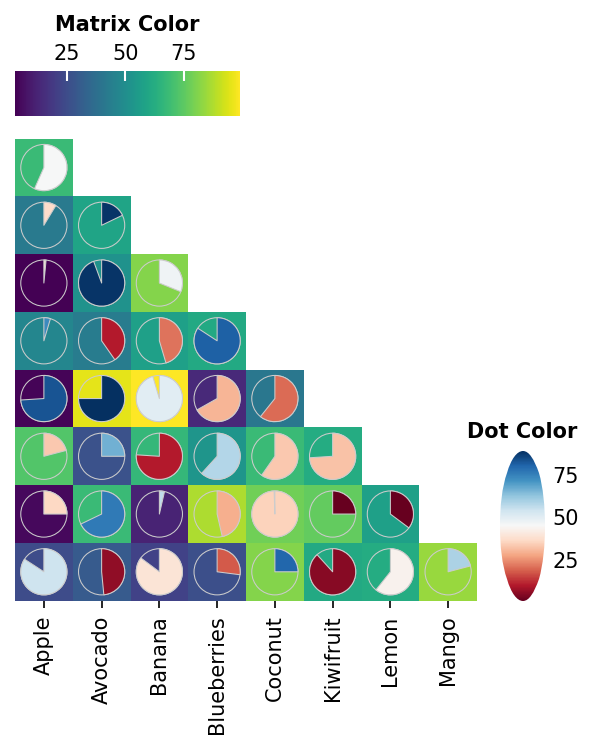# Triangle Dot heatmap Example#

Here shows how to draw a triangle dot heatmap and dot heatmap + matrix heatmap

```import numpy as np
import milkviz as mv
from milkviz import mask_triu
```

## First let’s create some random data#

```np.random.seed(0)
shape = (8, 8)
colors = np.random.randint(1, 100, shape)
sizes = np.random.randint(1, 100, shape)
matrix = np.random.randint(1, 100, shape)
labels = ["Apple", "Avocado", "Banana", "Blueberries",
"Coconut", "Kiwifruit", "Lemon", "Mango"]
```

## Using masked array to create triangle#

You can replace all unwanted values into NaN

```sizes = mask_triu(sizes)
mv.dot_heatmap(sizes, colors, matrix, xticklabels=labels, dot_patch="pie",
dot_hue_cbar_kw={"title": "Dot Color"},
matrix_cbar_kw={"title": "Matrix Color"}
)
```Out:

```<milkviz._dot_matrix.DotHeatmap object at 0x7fc33db99fd0>
```

Total running time of the script: ( 0 minutes 1.377 seconds)

Gallery generated by Sphinx-Gallery